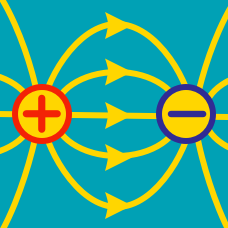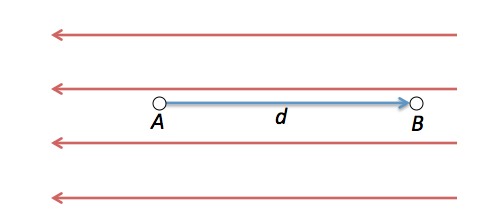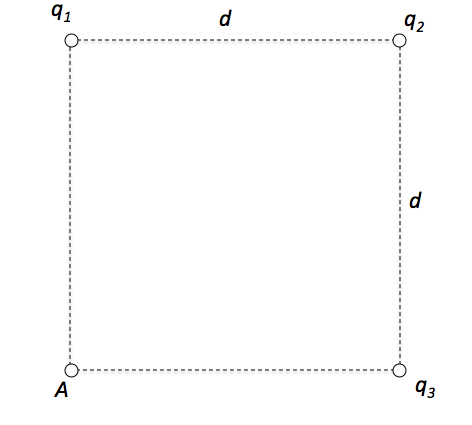Electricity and Magnetism

# Electric potential

Two large, parallel conducting plates are $d = 10 \text{ cm}$ apart and have charges of equal magnitude and opposite sign on their facing surfaces. An electric field of strength $E = 4.00 \times 10^4 \text{ N/C}$ acts on an electron placed anywhere between the two plates. (Neglect fringing.) What is the potential difference between the plates?A uniform electric field of $200 \text{ N/C}$ points to the left as shown in above figure. When the distance between points $A$ and $B$ is $d= 5 \text{ cm},$ what is the difference in potential $V_A - V_B$ between points $A$ and $B?$

A charge of $6.0 \text{ nC},$ is initially at a point that is $r_1 = 3.0 \text{ m},$ away from a charge of $1.0 \text{ nC}$ moves further away to a point where the distance is $r_2= 7.0 \text{ m}.$ What is the approximate potential difference between the two points.

Assume that electric constant is $\epsilon_0 = 8.9 \times 10^{-12} \text{ F/m}.$

A 9-V battery has an electric potential difference of $9\text{ V}$ between the positive and negative terminals. How much kinetic energy in J would an electron gain if it moved from the negative terminal to the positive one?

Details and Assumptions:

• The charge on the electron is $-1.6 \times 10^{-19}~\mbox{C}$.
• You may assume energy is conserved (so no drag or energy loss due to resistance for the electron).As shown in the above figure, three point charges $q_1 = 2.0 \text{ nC}, q_2 = 5.0 \text{ nC}$ and $q_3 = 4.0 \text{ nC}$ are placed at the three corners of a square with side length $d = 7 \text{ m}.$ Find the approximate potential at the point $A.$

Assume that electric constant is $\epsilon_0 = 8.9 \times 10^{-12} \text{ F/m}$ and $\sqrt{2}$ is $1.4.$

×